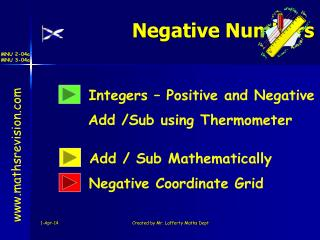DownloadDownload PresentationNegative Numbers

# Negative Numbers

Download Presentation## Negative Numbers

- - - - - - - - - - - - - - - - - - - - - - - - - - - E N D - - - - - - - - - - - - - - - - - - - - - - - - - - -
##### Presentation Transcript

1. Negative Numbers Integers – Positive and Negative Add /Sub using Thermometer www.mathsrevision.com Add / Sub Mathematically Negative Coordinate Grid Created by Mr. Lafferty Maths Dept

2. Starter Questions Q1. Calculate Q2. How many euros do I get for £20 if exchange rate is £1  1.5 € Q3. What is the time difference 09:28 and 10:50 www.mathsrevision.com Q4. The answer to the question is 90. What is the question. Created by Mr. Lafferty Maths Dept

3. Negative Numbers Addition & Subtraction Learning Intention Success Criteria • Understand how to add and subtract both positive and negative integers using a thermometer. • 1 We are learning how to add positive and negative numbers. www.mathsrevision.com Created by Mr. Lafferty Maths Dept

4. Integers Definition : An INTEGER is a negative or positive whole number, including 0. e.g. -2, -1, 0, 5, 100 Note : -2.5, -1.2, 23.5 Are NOT INTEGERS www.mathsrevision.com Write down 3 places where negative numbers are used Interpreting negative numbers Ex1 Page 59 Round Class Created by Mr. Lafferty Maths Dept

5. 24 22 20 18 16 14 12 10 UP + Down - -10 -12 -14 -16 -18 -20 -22 -24 Negative Numbers (a) 4oC up from 12 o C 8 6 12 + 4 = 16 4 (b) 8oC down from 10 o C 2 10 - 8 = 2 0 -2 (c) 3oC up from -2 o C -4 -6 -2 + 3 = 1 -8 www.mathsrevision.com (d) 6oC down from 2 o C 2 - 6 = -4 Created by Mr. Lafferty Maths Dept

6. Negative Numbers Your turn ! Page 61 Exercise 2 www.mathsrevision.com Created by Mr. Lafferty Maths Dept

7. Starter Questions Q1. Calculate Q2. How many euros do I get for £10 if exchange rate is £1  1.4 € Q3. What is the time difference 09:45 and 20:30 www.mathsrevision.com Q4. The answer to the question is 30. What is the question. Created by Mr. Lafferty Maths Dept

8. Negative Numbers Addition & Subtraction Learning Intention Success Criteria • To be able to write down mathematically the addition and subtraction of both positive and negative integers. • 1 We are learning how to write down mathematically addition and subtraction of negative integers. www.mathsrevision.com Created by Mr. Lafferty Maths Dept

9. 24 22 20 18 16 14 12 10 UP + Down - -10 -12 -14 -16 -18 -20 -22 -24 Negative Numbers (a) 12 + 4 = 16 8 6 4 Go up 4 Start at 12 2 0 -2 (b) 10 +(-8) = 2 -4 -6 -8 www.mathsrevision.com Start at 10 Go down 8 (c) 7 - 10= -3 Start at 7 Go down 10 Created by Mr. Lafferty Maths Dept

10. Negative Numbers Examples Remember “+ -” = - “- +” = - (1) 3 - 7 = -4 (2) 4 + (-9) = -5 (3) -7 - 8 = -15 www.mathsrevision.com (4) -2 + (-12) = -14 (5) -5 - 25 = -30 (6) 8 + (-9) = -1 Created by Mr. Lafferty Maths Dept

11. Negative Numbers Your turn ! Page 61 Exercise 2 Q1 and Q2 www.mathsrevision.com Created by Mr. Lafferty Maths Dept

12. Starter Questions www.mathsrevision.com Created by Mr. Lafferty Maths Dept

13. Coordinates Extending the Grid – Negative Numbers Learning Intention Success Criteria • Remember the main parts of a coordinate grid. • 1. We are learning how we can extend the coordinate grid to include negative numbers. 2. Understand the negative grid both in y-axis direction and x-axis direction. www.mathsrevision.com Created by Mr. Lafferty Maths Dept

14. Coordinates Extending the Grid – Negative Numbers We can further extend the negative grid y - axis Plot the point (-2,2) 3 x-coordinate is -2 2 1 y-coordinate is 2 www.mathsrevision.com 0 -2 -3 -1 2 4 -4 1 3 x - axis -1 (-4,-2) -2 -3 x-coordinate is -4 y-coordinate is -2 Write down coordinates of the other points Created by Mr. Lafferty Maths Dept

15. Coordinates Extending the Grid Now try Exercise 4 Ch5 (page 64) www.mathsrevision.com Created by Mr. Lafferty Maths Dept